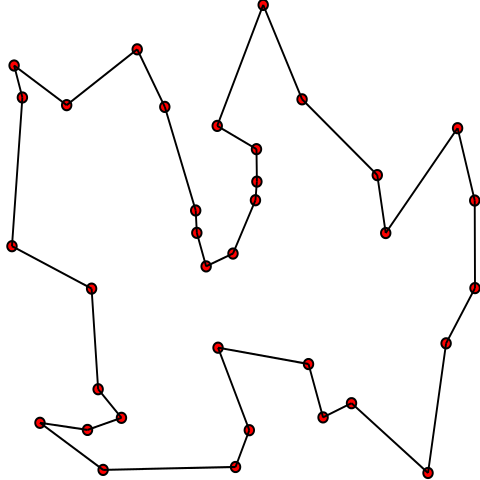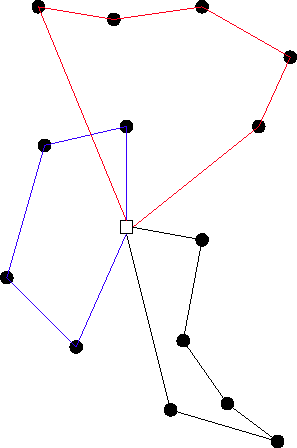## The Travelling Salesman Problem

### First NP-Hard Problem

Given a graph $\graph$, compute the shortest tour linking all nodes.\begin{align} \min && \sum_{i, j \in E} x_{i,j} & \cdot \cost_{i,j} \\ s.t. && \sum_{j \in C} x_{i,j} & = 1 & \forall i \in C \\ && \sum_{j \in C} x_{j,i} & = 1 & \forall i \in C \\ && \sum_{i, j \in S} x_{i,j} & \leq |S| - 1 & \forall S \subseteq C \\ && x \in \B \end{align}

## The Vehicle Routing Problem

### TSP with More than One Traveller

Given a graph $\graph$, a set of customer demands, and a set of identical vehicles $V$, compute the shortest routing so that all customers are visited once.\begin{align} \min && \sum_{v \in V} \sum_{i,j \in E} & x_{i,j}^v \cdot \cost_{i,j} \\ s.t. && \sum_{v \in V} y^v_i & = 1 & \forall i \in C \\ && \sum_{i \in C} y^v_i \cdot \dem_i & \leq \capa & \forall v \in V \\ && y^v_i & \leq \sum_{j \in C} x_{j,i}^v & \forall v \in V, i \in C \\ && \sum_{j \in C} (x_{j, i}^v - x_{i, j}^v) & = 0 & \forall v \in V \\ && \sum_{i, j \in S} x^v_{i,j} & \leq |S| - 1 & \forall S \subseteq C, v \in V \\ && x \in \mathbb{B}&, y \in \mathbb{B} \end{align}

## The Split Delivery Vehicle Routing Problem

### VRP with Multiple Visits

Relaxation of the VRP where customers can be visited more than once.

\begin{align} \min && \sum_{v \in V} \sum_{i,j \in E} & x_{i,j}^v \cdot \cost_{i,j} \\ s.t. && \color{red}{\sum_{v \in V} y^v_i} & \color{red}{= \dem_i} & \forall i \in C \\ && \sum_{i \in C} y^v_i & \leq \capa & \forall v \in V \\ && y^v_i & \leq \sum_{j \in C} x_{j,i}^v & \forall v \in V, i \in C \\ && \sum_{j \in C} (x_{j, i}^v - x_{i, j}^v) & = 0 & \forall v \in V \\ && \sum_{i, j \in S} x^v_{i,j} & \leq |S| - 1 & \forall S \subseteq C, v \in V \\ && x \in \mathbb{B}&, \color{red}{y \geq 0} \end{align}

## The Rich VRP

### Real World Complexity

• Based on a tender to optimise grocery delivery in Queensland
• First MIP model developed in  as a benchmark for comparison against CP
• Can be extended using valid cuts or symmetry breaking
• Fleet and routing can be modelled in numerous ways
• Composite model

 Fleet Design Optimisation From Historical Data Using Constraint Programming and Large Neighbourhood Search
Tommaso Urli and Philip Kilby
CP 2015

## The Rich VRP

### Core Model

Given a graph $\graph$, a set of vehicles $V$, a set of types $T$, and a set of commodities $K$. \begin{align} \min && \sum_{v \in V} \sum_{i,j \in E} x_{i,j}^v &\cdot \cost_{t} \cdot \mathbf{d}_{i,j} \\ s.t. && \sum_{v \in V} y_{i, k}^v & = \dem_{i, k} & \forall i \in C, k \in K \\ && \sum_{i \in C} \sum_{k \in K} y_{i, k}^v & \leq \capa_{t} & \forall v \in V \\ && u_{v} & \leq x_{i, i}^v & \forall i,j \in E \\ && x \in \mathbb{B}, u \in \mathbb{B}&, y \geq 0 \end{align}

## The Rich VRPs

### Flexible fleet

• We know the number of vehicles
• Type is a decision variable $z_t^v$
• Four-index routing $x_{t, i,j}^v$
• Compatibility needs to be explicit

### Stable Fleet

• Vehicles have types
• We know how many per type
• Three-index routing $x_{i,j}^v$
• Compatibility implicit

## The Rich VRPs

### Vehicle flow

• What enters must leave $$\sum_{j \in C} x_{i,j}^v = \sum_{j \in C} x_{j, i}^v$$
• Sub-tour elimination $$\sum_{i,j \in S} x_{i,j}^v \leq |S| - 1$$

### Commodity flow

• Variable to track the amount carried on an arc $f_{k, i,j}^{v}$
• Delivery handles sub-tours $$\sum_{k \in K} \sum_{j \in C} (f_{k,i,j}^{v} - f_{k,j,i}^{v}) = y_{i,k}^v$$

## The Rich VRP

### Valid Cuts

• Minimum visits
• Minimum vehicles
• Maximum vehicles
• Depot outgoing degree
• Single visit

### Symmetry Breaking

• Vehicle usage
• Visit order
• Fleet order
• Customer assignment

Warm-starting

## Experimental Setting

• Gurobi v6.5
• 15min runs
• Based on real data
• Three category of instances: small, medium, large

## Results

### MIP + Valid Cuts + Symmetry + Warm-Start

 sc sf fc ff 1 2 3 4 5 6 7 8 9 29.31 522.84 72.99 222.91 447.78 664.57 (0.45%) 4.58 160.73 27.82 806.01 664.07 207.41 (1.48%) (2.15%) (1.09%) 4.61 183.37 19.83 598.22 506.86 438.87 (1.37%) (1.10%) (1.53%) 27.21 161.06 58.84 (0.36%) (0.26%) 517.95 (1.65%) (3.51%) (3.16%) 18.78 438.17

## Results

### Using a Year of Data

 CP MIP CP (1min) + MIP Value Best (%) Diff (%) Value Best (%) Diff (%) Gap (%) Value Best (%) Diff (%) Gap (%) small 49303 0.83 0.95 48852 8.83 0.00 0.74 48850 90.34 0.01 0.79 medium 51698 7.73 3.83 51199 38.98 2.79 6.97 50446 53.29 1.46 6.12 large 52518 7.73 5.08 52200 26.25 4.17 9.85 50646 65.74 1.56 7.68